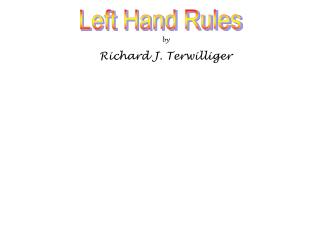DownloadDownload PresentationLeft Hand Rules

# Left Hand Rules

Download Presentation## Left Hand Rules

- - - - - - - - - - - - - - - - - - - - - - - - - - - E N D - - - - - - - - - - - - - - - - - - - - - - - - - - -
##### Presentation Transcript

1. Left Hand Rules by Richard J. Terwilliger

2. Left Hand Rule st Left Hand Rule 1 Left Hand Rule B Click on a i N LEFT HAND RULE S rd 3 nd 2 F Left Hand Rules Created by Richard J. Terwilliger July 2001

3. Left Hand Rule st Left Hand Rule 1 Left Hand Rule B i N S rd 3 nd 2 F Left Hand Rules

4. st 1 Left Hand Rule e e e e e e Magnetic Fields around current bearing wires

5. st 1 Left Hand Rule e e e e e e Thumb points in the direction of electron flow. Fingers curl around the wire in the direction of the magnetic field.

6. N The magnetic field in front of the wire points towards the top of the page.

7. N The magnetic field behind the wire points towards the bottom of the page.

8. N The magnetic field above the wire points into the page.

9. N The magnetic field below the wire points out of the page.

10. Again, The thumb of the left hand points in the direction of electron flow The fingers curl around the wire in the direction of the magnetic field

11. The magnetic field in front of the wire is to the left

12. The magnetic field behind the wire is to the right

13. The magnetic field on the right side of the wire is pointed out of the page

14. Out of the page is shown by a dot X Into the page is shown by an X

15. X Out of the page is shown by a dot X Into the page is shown by an X X

16. X Out of the page is shown by a dot X Into the page is shown by an X X

17. e e e e e e LEFT Grasp the wire with your hand The current flow is now to the left

18. e e e e e e The current flow is now to the left The thumb points in the direction of electron flow, the fingers curl around the wire in the direction of the magnetic field.

19. e e e e e e X X X X The current flow is now to the left The thumb points in the direction of electron flow, the fingers curl around the wire in the direction of the magnetic field.

20. e e e e e e The current flow is now to the left X X X X The thumb points in the direction of electron flow, the fingers curl around the wire in the direction of the magnetic field.

21. Each of the following diagrams shows a section of wire that has been enlarged. Associated with each wire is the direction of current flow and the magnetic field around the wire. Determine which of the following diagrams are correct.

22. Does the diagram at the right show the correct orientation of the magnetic field around the current bearing wire?. CORRECT WRONG

23. ? CORRECT WRONG Is the diagram at the left correct? X X X X X X The diagram is…

24. The diagram shows a compass placed above a current bearingwire. compass

25. The compass needle points into the page

26. What is the direction of the current flow in the wire? East West Click on your choice above.

27. Left Hand Rule nd 2

28. Loops Shown here is a loop of wire connected to a potential source.

29. negative Loops The electrons flow from the negative terminal of the battery

30. positive Loops through the wire and back to the positive terminal.

31. Loops through the wire and back to the positive terminal.

32. Loops We know that when current flows through a wire a magnetic field is formed.

33. We use the Left Hand Rule to determine the direction of the magnetic field. nd 2 Loops

34. Left Hand Rule nd 2 for Loops Coils and The arrows show the direction of electron flow. Fingers curl in the direction of the magnetic field

35. Grab the loop with your Left Hand Rule LEFT HAND nd 2 for Loops Coils and

36. Left Hand Rule nd 2 for Loops Coils and Curl your fingers around the loop in the same direction as the electron flow.

37. Left Hand Rule nd 2 for Loops Coils and Your thumb now points S NORTH N

38. S N The magnetic field on the outside of the loop is from the north pole to the south pole

39. S N The magnetic field inside the loop travels from the south back to the north

40. N S N If we place a compass inside the loop it points in the direction of the flux lines

41. N N N S N Outside the loop a compass still points in the direction of the magnetic flux lines

42. I’m back!

43. Several LOOPS of wire are called a Coil Coils

44. We also use the Left Hand Rule to determine the magnetic field around a coil. nd 2 Coils

45. To demonstrate the Left Hand Rule we’ll start by building an electromagnet. nd 2 Coils

46. To build an electromagnet or solenoid we start with a cylinder. Coils

47. We could use one of the cardboard rolls found at the center of toilet paper rolls Coils

48. If the inside of the cylinder is hollow it is said to have an air core. Air Core